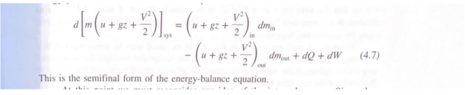# How to find velocity of gas pump flowing into car tank

• medale14

#### medale14

TL;DR Summary
Hello, so I am stuck with the concern of making an equation that fits my standard. I'm trying to create an equation from the mass, momentum and energy balance equation according to my system. My system is a gas pump flowing into a car tank. In this to make it easier for myself I disregarded friction. I understand that to solve this equation I must use the cross-sec area of the nozzle. However, I'm stuck with how to set up the rest of the equation. Would I take inconsideration p atm?
The general balance equation is as follow: d[m{u+gz+v2/2)sys=(u+gz+v2/2)dmin-(u+gz+v2/2)dmout +dq+dw.

I understand that I would take in consideration the cross sectional area as well as the mass flow rate. However I can't figure out what else to consider as if atm pressure should be considered and vapor pressure? My main goal is to figure out the velocity if the gas being pumped into the tank.

#### Attachments

•Screenshot 2022-10-13 183213.png
16.5 KB · Views: 66
Let's just pump water first. Vapor pressure for gasoline might be an issue but not now. So what are you tryimg to specify?
Pump pressure required to deliver a certain flow rate? Please specify exactly what you want to know

I'm just trying to figure out an equation that can help me figure out the velocity for pumping gas into a car tank.

I'm just trying to figure out an equation that can help me figure out the velocity for pumping gas into a car tank.
Can you please define all of your terms in your equation in your first post? And in the future, please try to post math in LaTeX (see the LaTeX Guide link in the Edit window). Thanks.